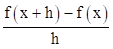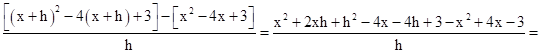# V-n graph

Classified in Mathematics

Written at onEnglish with a size of 10.22 KB.

parellel=orig equation      PP = - recipricol

x= -b(+-)SQROOT( b^2-4*a*c)/2*a, complex solutions     vtex= (negative) (-)b/2a

(x/2)^2                                                  if a > 0, then the parabola opens up

Parabola form = a(x-h)^2+k                                             if a < 0,="" then="" the="" parabola="" opens="">

P=2L+2W

x+x+z=y

A=L*W

N=odd and an>0= Falls Left + Rise right

N=odd and an<0= rise="" left="" +="" falls="">0=>

N=Even and an>0= Rise left + Rise Right

N=Even and an<0= falls="" left="" +="" falls="">0=>

Rational zero=P/Q

factor = x - zero

Quotient= Synthetic division

Distance = SQROOT((X2-X1)^2+(Y2-Y1)^2)

reflection about the x-axis of the graph y = x2 and y = -x2

Symmetry to the x-axis (x, -y) is on the graph when (x, y) is. Test for symmetry In the equation, leave x alone and replace y with -y. If you get the same equation, it is symmetric to the x-axis.

Symmetry to the y-axis (-x, y) is on the graph when (x, y) is. Test for symmetry. In the equation, replace x with -x. If the equation is the same, it is symmetric to tye y-axis.

Symmetry to the line y = x. (y, x) is on the graph when (x, y) is. Test for symmetry. Interchange x and y. If the equation is the same then it is symmetric to the line y = x.

Symmetry to the origin. (-x, -y) is on the graph when (x, y) is. Test for symmetry. Replace x with -x and y with -y. If the equation is the same, then it is symmetric to the origin.

If a zero has odd multiplicity, then the graph crosses the x-axis at the zero.

if a zero has even multiplicity, then the graph touches the -axis at the zero

if NM, no HA

Difference Quotienty=g(1/2x) divide by 1/2 or multiply by 2 = Horizontal           C>1 shrink     0<><1 stretch="">1>

y=1/2g(x) means Multiply by 1/2 = Vertical                            C>1 stretch     0<><1 shrink="">1>

Linear Function: f(x) = mx + b = slanted line

Square Function: f(x) = x^2 = U

Cube Function: f(x) = x^3 = Z

Square Root Function: f(x) = SQROOT(x) = Hook

Absolute Value Function: f(x) = |x| = V

Reciprocal Function: f(x) = 1/x = >

The Cube Root Function: f(x) = = S

Step 1: Enter the data Calculator: STAT, EDIT Enter the two columns of data. Note: To clear old data from a column quickly, move the cursor to the top of the column (on top of L1 or L2, etc.), hit CLEAR and then ENTER.

Step 2: Find the line of regression Calculator: STAT, arrow right to CALC, arrow down to #4 (LinReg (ax + b)), ENTER or CALCULATE (to return to the home screen), and ENTER again to calculate. Important Note: When calculating the line of regression, we are sometimes asked to find the correlation coefficient, denoted with an r. We need to set up the calculator so that it will show r. You should only need to do this on your calculator once, but you may also need to do it on any calculator you use in the test center. Turning on the correlation coefficient: 1. Hit the “Catalog” key on your calculator (2nd , the number zero). 2. Scroll down to “Diagnostic On” 3. Hit “Enter” and it should appear on the homescreen. 4. Hit “Enter” again, and you are done. Remember: The closer the r is to 1 or ─1, the closer the data fits a line.

,>